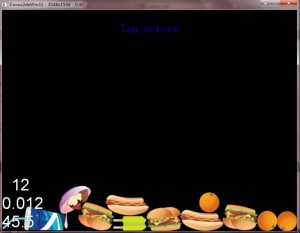# cocos2d-x读取PhisicalEditor输出文件```CCArray *fixtureList = (CCArray *)(bodyData->objectForKey("fixtures"));
FixtureDef **nextFixtureDef = &(bodyDef->fixtures);
//<ObjectDict *>
int con = fixtureList->count();
for (int i = 0; i<con; i++) {
b2FixtureDef basicData;
ObjectDict *fixtureData = (ObjectDict *) fixtureList->objectAtIndex(i);

basicData.filter.categoryBits = static_cast<CCString *>(fixtureData->objectForKey("filter_categoryBits"))->intValue();
basicData.filter.groupIndex = static_cast<CCString *>(fixtureData->objectForKey("filter_groupIndex"))->intValue();
basicData.friction = static_cast<CCString *>(fixtureData->objectForKey("friction"))->floatValue();
basicData.density = static_cast<CCString *>(fixtureData->objectForKey("density"))->floatValue();
basicData.restitution = static_cast<CCString *>(fixtureData->objectForKey("restitution"))->floatValue();
basicData.isSensor = (bool)static_cast<CCString *>(fixtureData->objectForKey("isSensor"))->intValue();

CCString *cb = static_cast<CCString *>(fixtureData->objectForKey("userdataCbValue"));

int callbackData = 0;```

```CCArray* CCDictionary::allKeys()
{
int iKeyCount = this->count();
if (iKeyCount <= 0) return NULL;

CCArray* pArray = CCArray::createWithCapacity(iKeyCount);

CCDictElement *pElement, *tmp;
if (m_eDictType == kCCDictStr)
{
HASH_ITER(hh, m_pElements, pElement, tmp)
{
CCString* pOneKey = new CCString(pElement->m_szKey);
pOneKey->autorelease();
}
}
else if (m_eDictType == kCCDictInt)
{
HASH_ITER(hh, m_pElements, pElement, tmp)
{
CCInteger* pOneKey = new CCInteger(pElement->m_iKey);
pOneKey->autorelease();
}
}

return pArray;
}```

```CCDictElement* cde = NULL;
CCDICT_FOREACH(m_itemlist,cde){
}```